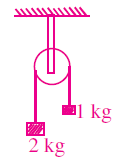Two blocks of masses 1 kg and 2kg are connected by a metal wire going over a smooth pulley as shown. The breaking stress of the metal is 403π x 106 Nm-2. If g = 10ms–2. Then what should be the minimum radius of the wire used if it is not to break?

# Two blocks of masses 1 kg and 2kg are connected by a metal wire going over a smooth pulley as shown. The breaking stress of the metal is . If g = 10ms–2. Then what should be the minimum radius of the wire used if it is not to break?1. A

0.5mm

2. B

2mm

3. C

1mm

4. D

1.5mm

Fill Out the Form for Expert Academic Guidance!l

+91

Live ClassesBooksTest SeriesSelf Learning

Verify OTP Code (required)

### Solution:

$⇒$r2 = 10-6 $⇒$ r = 10–3 $⇒$ r = 1mm

## Related content

 Differences & Comparisons Articles in Physics Important Topic of Physics: Reynolds Number Distance Speed Time Formula Refractive Index Formula Mass Formula Electric Current Formula Electric Power Formula Resistivity Formula Weight Formula Linear Momentum Formula+91

Live ClassesBooksTest SeriesSelf Learning

Verify OTP Code (required)